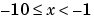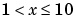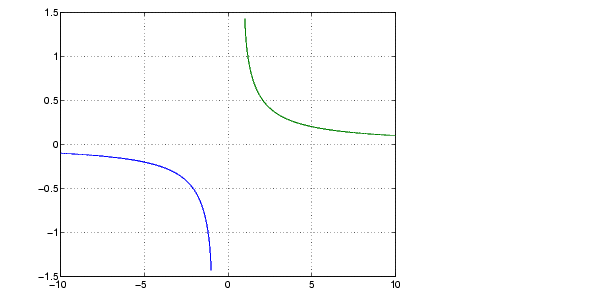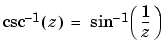MATLAB Function Referenceacsc

Inverse cosecant, result in radians

Syntax

• ```Y = acsc(X)
```

Description

```Y = acsc(X) ``` returns the inverse cosecant (arccosecant) for each element of `X`.

The `acsc` function operates element-wise on arrays. The function's domains and ranges include complex values. All angles are in radians.

Examples

Graph the inverse cosecant over the domainsand.

• ```x1 = -10:0.01:-1.01;
x2 = 1.01:0.01:10;
plot(x1,acsc(x1),x2,acsc(x2)), grid on```

Definition

The inverse cosecant can be defined as

•Algorithm

`acsc` uses FDLIBM, which was developed at SunSoft, a Sun Microsystems, Inc. business, by Kwok C. Ng, and others. For information about FDLIBM, see http://www.netlib.org.

See Also

`csc`, `acscd`, `acsch`

© 1994-2005 The MathWorks, Inc.